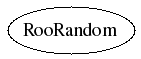library: libRooFit#include "RooRandom.h"

# RooRandom

class description - header file - source file

## class RooRandom### Function Members (Methods)

Display options:
Show inherited
Show non-public
public:
 RooRandom(const RooRandom&) virtual ~RooRandom() static TClass* Class() static Double_t gaussian(TRandom* generator = randomGenerator()) static UInt_t integer(UInt_t max, TRandom* generator = randomGenerator()) virtual TClass* IsA() const RooRandom& operator=(const RooRandom&) static Bool_t quasi(UInt_t dimension, Double_t* vector, RooQuasiRandomGenerator* generator = quasiGenerator()) static RooQuasiRandomGenerator* quasiGenerator() static TRandom* randomGenerator() virtual void ShowMembers(TMemberInspector& insp, char* parent) virtual void Streamer(TBuffer& b) void StreamerNVirtual(TBuffer& b) static Double_t uniform(TRandom* generator = randomGenerator()) static void uniform(UInt_t dimension, Double_t* vector, TRandom* generator = randomGenerator())
private:

## Class Description

``` Return a pointer to a singleton random-number generator
implementation. Creates the object the first time it is called.
```

``` Return a pointer to a singleton quasi-random generator
implementation. Creates the object the first time it is called.
```
Double_t uniform(TRandom *generator)
``` Return a number uniformly distributed from (0,1)
```
void uniform(UInt_t dimension, Double_t vector[], TRandom *generator)
``` Fill the vector provided with random numbers uniformly distributed from (0,1)
```
UInt_t integer(UInt_t n, TRandom *generator)
``` Return an integer uniformly distributed from [0,n-1]
```
Double_t gaussian(TRandom *generator)
``` Return a Gaussian random variable with mean 0 and variance 1.
```
Bool_t quasi(UInt_t dimension, Double_t vector[], RooQuasiRandomGenerator *generator)
``` Return a quasi-random number in the range (0,1) using the
Niederreiter base 2 generator described in Bratley, Fox, Niederreiter,
ACM Trans. Model. Comp. Sim. 2, 195 (1992).
```
virtual ~RooRandom()
`{}`

Last update: Sat Dec 9 09:59:40 2006
Copyright (c) 2000-2005, Regents of the University of California *

ROOT page - Class index - Class Hierarchy - Top of the page

This page has been automatically generated. If you have any comments or suggestions about the page layout send a mail to ROOT support, or contact the developers with any questions or problems regarding ROOT.